Published

# Portable Electrocardiograph (ECG)

A step by step tutorial that will guide you throught the complete development process. It also includes prototype testing. A success!

IntermediateFull instructions provided3 days18,380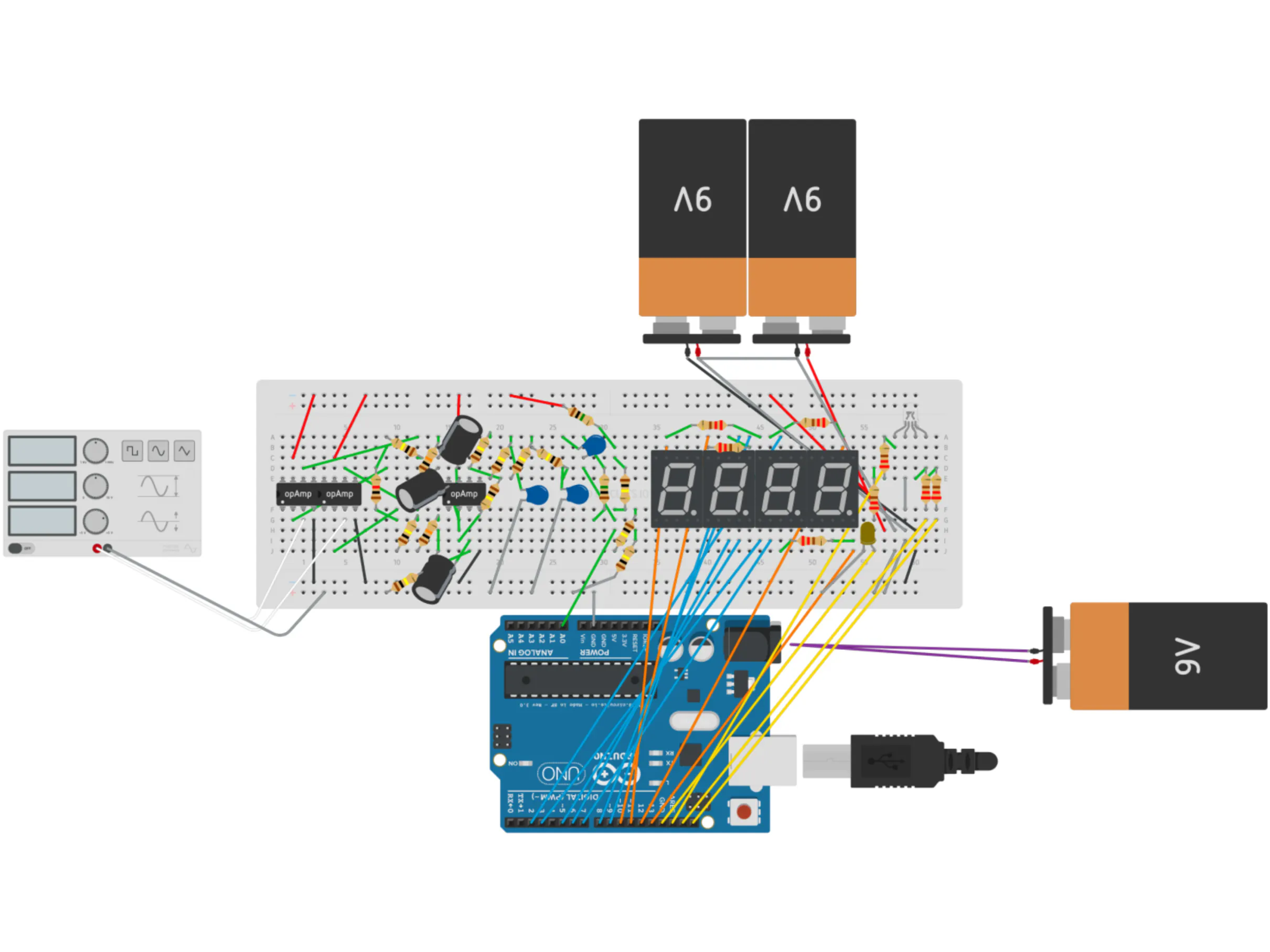## Things used in this project

### Hardware components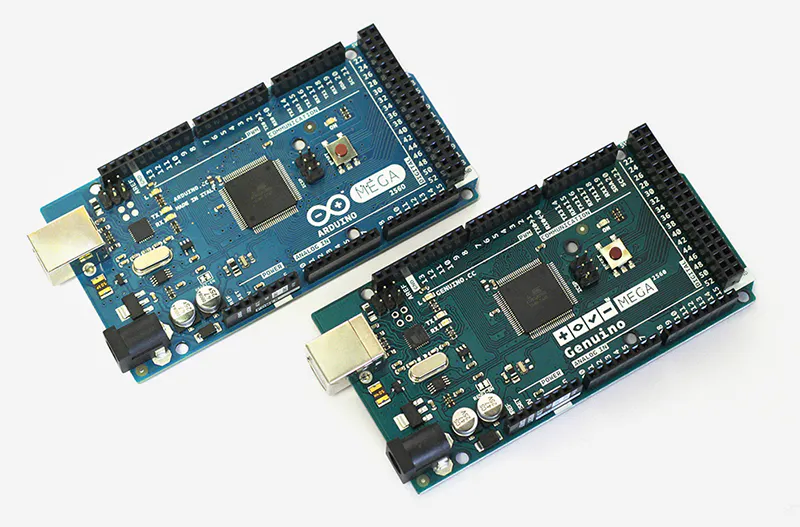Arduino Mega 2560 & Genuino Mega 2560
×1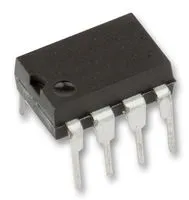Texas Instruments General Purpose Dual Op-Amp
×3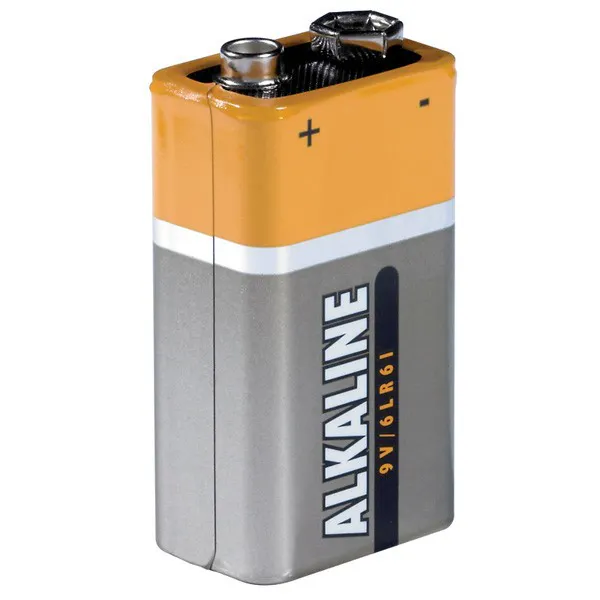9V battery (generic)
×3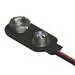9V Battery Clip
×3
 Tiga-Med Gold Electrodes
×3
 Capacitor 18nF
×1
 Capacitor 33nF
×1
 Capacitor 1uF
×1
 Capacitor 10uF
×1
 Capacitor 20 uF
×1Resistor 1k ohm
×1Resistor 10k ohm
×2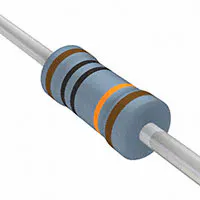Resistor 100k ohm
×4
 Resistor 200k ohm
×3
 Resistor 1.1M ohm
×1
 Resistor 3M ohm
×1

### Software apps and online servicesArduino IDE
 PartSim
 Autodesk Circuits

### Hand tools and fabrication machinesSoldering iron (generic)

## Code

### Digital Filters

MATLAB
A IIR notch and Butterwoth high-pass filter.
`No preview (download only).`

### ECG - Plot

Arduino
ECG code along with respective curve's visualization.
```// -------------------------------------- Parameters ------------------------------------

int ledPin = 50;
int ledPin2 = 48;
int ledPin3 = 46;
int ledPin4 = 44;
int ledPin5 = 13;

// Originally collected signal (x, x1 and x2), notch filtered (y, y1, y2 and y3) and notch & low-pass filtered ECG signal (z, z1, z2 and z3).
// x = x(t); x1 = x(t-1); x1 = x(t-2). The same applies for y and z.
volatile double x = 0.0;
volatile double x1 = 0.0;
volatile double x2 = 0.0;

volatile double y = 0.0;
volatile double y1 = 0.0;
volatile double y2 = 0.0;
volatile double y3 = 0.0;

volatile double z = 0.0;
volatile double z1 = 0.0;
volatile double z2 = 0.0;
volatile double z3 = 0.0;

String bpm = "Normal Heart Rate";

double maxi;
double mini;

// Flag variable.
int aux = 0;

// Iterative variable.

int ii = 0;
int k = 0;
int l;

// Arrays
double timeVecto2 ; // Array which keeps 512 samples from the ECG signal (more or less, one cycle: sampling frequency = 500 Hz)
double timeVectorHigh2 ; // Saves 60 different instants (in ms) which corresponds to each R peak in the signal.
double res1;
double res2;
double resf;
double maxx = 0;
double minn = 10000;

// Defining combination of on and off pins to show each number.
const int numeral = {
B11111100, // 0
B01100000, // 1
B11011010, // 2
B11110010, // 3
B01100110, // 4
B10110110, // 5
B10111110, // 6
B11100000, // 7
B11111110, // 8
B11110110, // 9
};

// Arduino pins used to connect all the 7 segments + dots.
const int segmentPins[] = { 22, 7, 8, 6, 5, 3, 2, 9};

// Number of digits allowed in the display.
const int numberofDigits = 4;

// Arduino pins used to connect all the 4 digits.
const int digitPins[numberofDigits] = {10, 11, 12, 4};
// --------------------------------------------------------------------------------------------------------- SETUP ---------------------------------------------------------------------------------------------------------

void setup() {

Serial.begin(9600);

pinMode(ledPin, OUTPUT);
pinMode(ledPin2, OUTPUT);
pinMode(ledPin3, OUTPUT);
pinMode(ledPin4, OUTPUT);
pinMode(ledPin5, OUTPUT);

for (int i = 0; i < 8; i++)
pinMode(segmentPins[i], OUTPUT); //set segment and DP pins to output

//sets the digit pins as outputs
for (int i = 0; i < numberofDigits; i++)
pinMode(digitPins[i], OUTPUT);

cli(); //stop interrupts

//set timer1 interrupt at 500Hz
TCCR1A = 0;// set entire TCCR1A register to 0
TCCR1B = 0;// same for TCCR1B
TCNT1  = 0;//initialize counter value to 0
// set compare match register for 1Hz increments
OCR1A = 3999;// = (16*10^6) / (8*500) - 1 (must be <65536)
// turn on CTC mode
TCCR1B |= (1 << WGM12);
// Set CS11 bits for 8 prescaler
TCCR1B |= (1 << CS11);
// enable timer compare interrupt
TIMSK1 |= (1 << OCIE1A);

sei(); //allow interrupts

}

// ------------------------------------------------------------------------------------------------------ INTERRUPTOR ------------------------------------------------------------------------------------------------------

ISR(TIMER1_COMPA_vect) { // commands executed at each time interrupt

// -------------------------------------------- Acquiring Data ------------------------------------------

// ----------------------------------------------- Filtering --------------------------------------------

// Filtering around 40 and 60 Hz (notch).
y = 0.2012 * x - 0.3256 * x1 + 0.2012 * x2 + 0.3256 * y1 + 0.5975 * y2;

// Low-pass Filter 100 Hz.
z = 0.0495 * y + 0.1486 * y1 + 0.1486 * y2 + 0.0495 * y3 + 1.1619 * z1 - 0.6959 * z2 + 0.1378 * z3;

x2 = x1;
x1 = x;

y3 = y2;
y2 = y1;
y1 = y;

z3 = z2;
z2 = z1;
z1 = z;

// Saving z value
timeVecto2[k] = z;

k = (k + 1) % 512;

}

// --------------------------------------------------------------------------------------------------------- LOOP ---------------------------------------------------------------------------------------------------------

void loop() {

// ------------------------------------------ Plotting ECG Curve ----------------------------------------

Serial.print(5 * 1000 * z / 1023);

Serial.println();

// ----------------------------------------- Detecting Heart Beat ---------------------------------------
maxi = 0;
mini = 10000;

showNumber(round(resf));

for (l = 0; l < 512; l++) {

if (maxi < timeVecto2[l]) {

maxi = timeVecto2[l]; // Signal Maximum's amplitude detected during one cycle.

}

if (mini > timeVecto2[l]) {

mini = timeVecto2[l]; // Signal Minimum's amplitude detected during one cycle.

}

}

// ----------------------------------------- Detecting Heart Beat ---------------------------------------

//

if (maxi - mini < 400 && maxi - mini > 150) {

digitalWrite(ledPin4, HIGH);

} else {

digitalWrite(ledPin4, LOW);
}

// DETECTING R PEAK.

if ( z > 0.9 * maxi ) {

if (aux == 0) {

timeVectorHigh2[ii] = millis(); // Saving 30 R peak time instants.

aux = 1;

res1 = 60000 / (timeVectorHigh2[ii] - timeVectorHigh2[(ii - 1) % 60]);

res2 = 60000 * 5 / (timeVectorHigh2[ii] - timeVectorHigh2[(ii - 5) % 60]);

ii = (ii + 1) % 60;

}
digitalWrite(ledPin5, HIGH);   // sets the LED on.
} else {
digitalWrite(ledPin5, LOW);    // sets the LED off.
aux = 0;
}

// ---------------------------------------- Calculating Heart Rate --------------------------------------

if (res1 > 40 && res1 < 200 && res2 > 40 && res2 < 200) {

if (ii > 2) {

resf = 0.5 * res1 + 0.5 * res2; // Weighting heart rate shown in the 4 digits 7 segments display. 30 % to Instant heart rate and 70 % to an average along 30 peaks to stabilize the output value.

}

}

// ----------------------------------- Detecting Unusual Conditions ---------------------------------

// DETECTING SINUS HEART RATE (beats per minute - bpm).

if (resf < 60) {

digitalWrite(ledPin, LOW);
digitalWrite(ledPin2, HIGH);
digitalWrite(ledPin3, HIGH);

} else if (resf >= 60 && resf < 100) {

bpm = "Normal Heart Rate";

digitalWrite(ledPin2, LOW);
digitalWrite(ledPin, HIGH);
digitalWrite(ledPin3, HIGH);

} else {

bpm = "Sinus Tachycardia";

digitalWrite(ledPin3, LOW);
digitalWrite(ledPin, HIGH);
digitalWrite(ledPin2, HIGH);

}

}

// -------------------------- 4 Digits 7 Segment Display (Auxiliar Functions) ------------------------

void showNumber (int number) {

if (number == 0)
showDigit (0, numberofDigits - 1); //display 0 in the rightmost digit.

else {

for (int digit = numberofDigits - 1; digit >= 0; digit--) {

if (number > 0) {

showDigit(number % 10, digit);

number = number / 10;

}

}

}

}

//Displays given number on a 7-segment display at the given digit position.
void showDigit (int number, int digit) {

digitalWrite(digitPins[digit], HIGH);

for (int segment = 1; segment < 8; segment++) {

isBitSet = ! isBitSet; // Remove this line if common cathode display.

digitalWrite(segmentPins[segment], isBitSet);

}

delay(5);

digitalWrite(digitPins[digit], LOW);

}
```

### ECG - Print

Arduino
ECG code along with respective printing.
```// -------------------------------------- Parameters ------------------------------------

int ledPin = 50;
int ledPin2 = 48;
int ledPin3 = 46;
int ledPin4 = 44;
int ledPin5 = 13;

// Originally collected signal (x, x1 and x2), notch filtered (y, y1, y2 and y3) and notch & low-pass filtered ECG signal (z, z1, z2 and z3).
// x = x(t); x1 = x(t-1); x1 = x(t-2). The same applies for y and z.
volatile double x = 0.0;
volatile double x1 = 0.0;
volatile double x2 = 0.0;

volatile double y = 0.0;
volatile double y1 = 0.0;
volatile double y2 = 0.0;
volatile double y3 = 0.0;

volatile double z = 0.0;
volatile double z1 = 0.0;
volatile double z2 = 0.0;
volatile double z3 = 0.0;

String bpm = "Normal Heart Rate";

double maxi;
double maxi2;
double mini;
double sum;

// Flag variables.
int aux = 0;
int aux2 = 0;
int aux3 = 0;
double auxx;

// Iterative variable.
int ii = 0;
int k = 0;
int l;

// Arrays
double timeVecto2 ; // Array which keeps 512 samples from the ECG signal (more or less, one cycle: sampling frequency = 500 Hz)
double timeVectorHigh2 ; // Saves 30 different instants (in ms) which corresponds to each R peak in the signal.
double timeVectorHigh3 ; // Time duration between 30 different R peaks.
double timeVectorLow2 ; // Saves 30 different instants (in ms) which corresponds to each S peak in the signal.
double timeVector2Medium ; // Saves 30 different instants (in ms) which corresponds to each T peak in the signal.
double rt ; // 30 different RT intervals from the ECG signal.
double rs; // 30 different RS intervals from the ECG signal.
double st; // 30 different ST intervals from the ECG signal.
double rs2;
double st2;
double rsf;
double stf;
double res1;
double res2;
double resf;
double maxx = 0;
double minn = 10000;

// Defining combination of on and off pins to show each number.
const int numeral = {
B11111100, // 0
B01100000, // 1
B11011010, // 2
B11110010, // 3
B01100110, // 4
B10110110, // 5
B10111110, // 6
B11100000, // 7
B11111110, // 8
B11110110, // 9
};

// Arduino pins used to connect all the 7 segments + dots.
const int segmentPins[] = { 22, 7, 8, 6, 5, 3, 2, 9};

// Number of digits allowed in the display.
const int numberofDigits = 4;

// Arduino pins used to connect all the 4 digits.
const int digitPins[numberofDigits] = {10, 11, 12, 4};
// --------------------------------------------------------------------------------------------------------- SETUP ---------------------------------------------------------------------------------------------------------

void setup() {

Serial.begin(9600);

pinMode(ledPin, OUTPUT);
pinMode(ledPin2, OUTPUT);
pinMode(ledPin3, OUTPUT);
pinMode(ledPin4, OUTPUT);
pinMode(ledPin5, OUTPUT);

for (int i = 0; i < 8; i++)
pinMode(segmentPins[i], OUTPUT); //set segment and DP pins to output

//sets the digit pins as outputs
for (int i = 0; i < numberofDigits; i++)
pinMode(digitPins[i], OUTPUT);

cli(); //stop interrupts

//set timer1 interrupt at 500Hz
TCCR1A = 0;// set entire TCCR1A register to 0
TCCR1B = 0;// same for TCCR1B
TCNT1  = 0;//initialize counter value to 0
// set compare match register for 1Hz increments
OCR1A = 3999;// = (16*10^6) / (8*500) - 1 (must be <65536)
// turn on CTC mode
TCCR1B |= (1 << WGM12);
// Set CS11 bits for 8 prescaler
TCCR1B |= (1 << CS11);
// enable timer compare interrupt
TIMSK1 |= (1 << OCIE1A);

sei(); //allow interrupts

}

// ------------------------------------------------------------------------------------------------------ INTERRUPTOR ------------------------------------------------------------------------------------------------------

ISR(TIMER1_COMPA_vect) { // commands executed at each time interrupt

// -------------------------------------------- Acquiring Data ------------------------------------------

// ----------------------------------------------- Filtering --------------------------------------------

// Filtering around 40 and 60 Hz (notch).
y = 0.2012 * x - 0.3256 * x1 + 0.2012 * x2 + 0.3256 * y1 + 0.5975 * y2;

// Low-pass Filter 100 Hz.
z = 0.0495 * y + 0.1486 * y1 + 0.1486 * y2 + 0.0495 * y3 + 1.1619 * z1 - 0.6959 * z2 + 0.1378 * z3;

x2 = x1;
x1 = x;

y3 = y2;
y2 = y1;
y1 = y;

z3 = z2;
z2 = z1;
z1 = z;

// Saving z value
timeVecto2[k] = z;

k = (k + 1) % 512;

}

// --------------------------------------------------------------------------------------------------------- LOOP ---------------------------------------------------------------------------------------------------------

void loop() {

// ------------------------------------------ Plotting ECG Curve ----------------------------------------

//  Serial.print(" ");
//  Serial.print(5 * 1000 * z / 1023);

//  Serial.println();

// ----------------------------------------- Detecting Heart Beat ---------------------------------------
maxi = 0;
maxi2 = 0;
mini = 10000;

showNumber(round(resf));

for (l = 0; l < 512; l++) {

if (maxi < timeVecto2[l]) {

maxi = timeVecto2[l]; // Signal Maximum's amplitude detected during one cycle.

}

if (mini > timeVecto2[l]) {

mini = timeVecto2[l]; // Signal Minimum's amplitude detected during one cycle.

}

if (timeVecto2[l] < (maxi + mini) / 2 && timeVecto2[l] - timeVecto2[(l - 2)%512] > 0 && timeVecto2[l] - timeVecto2[(l - 2)%512] < 2 && abs(timeVecto2[l] - timeVecto2[(l - 10)%512]) < 5 ) {

maxi2 = timeVecto2[l]; // Emprirical treshold for T peak detection.

}

}

// ----------------------------------------- Detecting Heart Beat ---------------------------------------

if (maxi - mini < 400 && maxi - mini > 100) {

digitalWrite(ledPin4, HIGH);

} else {

digitalWrite(ledPin4, LOW);
}

// DETECTING R PEAK.

if ( z > 0.9 * maxi ) {

if (aux == 0) {

timeVectorHigh2[ii] = millis(); // Saving 30 R peak time instants.
timeVectorHigh3[(ii - 1)%60] = timeVectorHigh2[ii] - timeVectorHigh2[(ii - 1)%60];

aux = 1;

res1 = 60000 / timeVectorHigh3[(ii - 1)%60];

res2 = 60000 * 5 / (timeVectorHigh2[ii] - timeVectorHigh2[(ii-5)%60]);

// Print of the acquired data
Serial.print("tS: ");
Serial.print( timeVectorLow2[ii]);
Serial.print("  ");
Serial.print("tT: ");
Serial.print( auxx);
Serial.print("  ");
Serial.print("tRS: ");
Serial.print( rs[ii]);
Serial.print("  ");
Serial.print("tST: ");
Serial.print( st[ii]);
Serial.print("  ");
Serial.print(" HR");
Serial.print( res1);
Serial.print("  ");

Serial.println();
Serial.print("ii: ");
Serial.print( ii);
Serial.print("  ");
Serial.print("tR: ");
Serial.print( timeVectorHigh2[ii]);
Serial.print("  ");

ii = (ii + 1) % 60;
}
digitalWrite(ledPin5, HIGH);   // sets the LED on.
} else {
digitalWrite(ledPin5, LOW);   // sets the LED off.
aux = 0;
}

// DETECTING S PEAK.

if ( z < 1.3 * (mini)) {

if(aux2 == 0){
timeVectorLow2[ii] = millis(); // Saving 30 S peak time instants.
//      Serial.print("tS: ");
//      Serial.print( timeVectorLow2[ii]);
//      Serial.print(" ");

aux2 = 1;
}

} else {

if(timeVectorLow2[ii] == 0){
timeVectorLow2[ii] = timeVectorLow2[(ii-1)%60];
}

aux2 = 0;
}

//Serial.print(rs);
//Serial.println();

// DETECTING T PEAK.

if ( z > 0.95*maxi2 && z < 0.8*maxi) {

auxx = millis();

if (aux3 == 0 && (auxx - timeVectorHigh2[(ii-1)%60]) > 200) {

timeVector2Medium[ii] = auxx;
//      Serial.print("tT: ");
//      Serial.print( auxx);
//      Serial.print(" ");
//      aux3 = 1;
}
aux3 = 1;

} else {

if(timeVector2Medium[ii] == 0){
timeVector2Medium[ii] = timeVector2Medium[(ii-1)%60];
}

aux3 = 0;
}

// ---------------------------------------- Calculating Heart Rate --------------------------------------

if (res1 > 40 && res1 < 200 && res2 > 40 && res2 < 200) {

resf = 0.5 * res1 + 0.5 * res2; // Weighting heart rate shown in the 4 digits 7 segments display. 50 % to Instant heart rate and 50 % to an average along 5 peaks to stabilize the output value.
}

// ----------------------------------- Detecting Unusual Conditions ---------------------------------

// DETECTING SINUS HEART RATE (beats per minute - bpm).

if (resf < 60) {

digitalWrite(ledPin, LOW);
digitalWrite(ledPin2, HIGH);
digitalWrite(ledPin3, HIGH);

} else if (resf >= 60 && resf < 100) {

bpm = "Normal Heart Rate";

digitalWrite(ledPin2, LOW);
digitalWrite(ledPin, HIGH);
digitalWrite(ledPin3, HIGH);

} else {

bpm = "Sinus Tachycardia";

digitalWrite(ledPin3, LOW);
digitalWrite(ledPin, HIGH);
digitalWrite(ledPin2, HIGH);

}

// Serial.print(bpm);
// Serial.print('\n');

// DETECTING SINUS ARRHYTHMIA

if (timeVectorHigh3[(ii - 2)%60] > maxx && ii > 1 && timeVectorHigh3[(ii - 2)%60] < 1200) {

maxx = timeVectorHigh3[(ii - 2)%60];

}

if (timeVectorHigh3[(ii - 2)%60] < minn && ii > 1 && timeVectorHigh3[(ii - 2)%60] > 320) {

minn = timeVectorHigh3[(ii - 2)%60];

}

if (maxx - minn > 160 && maxx - minn < 300 && bpm == "Sinus Tachycardia") {

//    Serial.print("Arrhythmia");
//    Serial.println();

} else {

//    Serial.print("Normal Heart Rate");
//    Serial.println();

}

// ----------------------------------- Showing Heart Rate Over Time ---------------------------------

//Serial.println(resf);

// ----------------------------------- RS Interval ---------------------------------

if (abs(timeVectorHigh2 [(ii - 1) % 60] - timeVectorLow2[ii]) < 120) {

rs [ii] = abs(timeVectorHigh2 [(ii - 1) % 60] - timeVectorLow2[ii]);

sum = 0;

for (l = 0; l < 60; l++) {

sum += rs[l];

}

rs2 = sum / 60;

rsf = 0.3 * rs2 + 0.7 * rs[ii];

} else {

rs [ii] = rs[(ii - 1) % 60];

}

// ----------------------------------- ST Interval ---------------------------------

if (abs(timeVector2Medium[ii] - timeVectorLow2[ii]) < 350 && abs(timeVector2Medium[ii] - timeVectorLow2[ii]) > 100) {

st [ii] = abs(timeVector2Medium [ii] - timeVectorLow2[ii]);

sum = 0;

for (l = 0; l < 60; l++) {

sum += st[l];

}

st2 = sum / 60;
stf = 0.3 * st2 + 0.7 * st[ii];

} else {

st [ii] = st[(ii - 1) % 60];

}

}

// -------------------------- 4 Digits 7 Segment Display (Auxiliar Functions) ------------------------

void showNumber (int number) {

if (number == 0)
showDigit (0, numberofDigits - 1); //display 0 in the rightmost digit.

else {

for (int digit = numberofDigits - 1; digit >= 0; digit--) {

if (number > 0) {

showDigit(number % 10, digit);

number = number / 10;

}

}

}

}

//Displays given number on a 7-segment display at the given digit position.
void showDigit (int number, int digit) {

digitalWrite(digitPins[digit], HIGH);

for (int segment = 1; segment < 8; segment++) {

isBitSet = ! isBitSet; // Remove this line if common cathode display.

digitalWrite(segmentPins[segment], isBitSet);

}

delay(5);

digitalWrite(digitPins[digit], LOW);

}
```

## Credits

### Luís Rita

3 projects • 26 followers
Biomedical Engineer. Data science student at Imperial College London. Passionate about science, sports and art.
Thanks to Gonçalo Frazão.